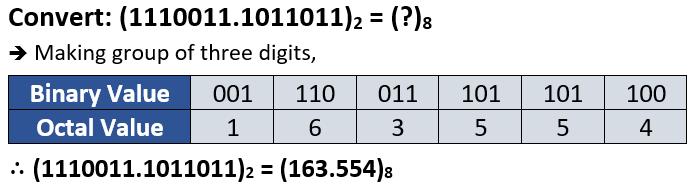Binary to Octal Conversion1. Binary Number is converted to Octal Number by making group of 3 BITS(Digits).
2. The grouping should be done from right to left for integer part and left to right for fraction part.
3. If there are less than 3 BITS in the last group, then the required number of zeros is added in the left hand side for the integer part and in the right hand side for the fraction part respectively to make the group of 3 BITS.
Then, equivalent octal value of each group is written as shown below.
Example 1;Example 2;Check the equivalent values of digits in different number system from here.